# Plus One Physics Notes Chapter 11 Thermal Properties of Matter

Students can Download Chapter 11 Thermal Properties of Matter Notes, Plus One Physics Notes helps you to revise the complete Kerala State Syllabus and score more marks in your examinations.

## Kerala Plus One Physics Notes Chapter 11 Thermal Properties of Matter

Summary
Temperature And Heat
Temperature is a measure of hotness of a body. Heat is a form of energy transferred between two system (or system and surrounding) by virtue of temperature difference. The SI unit of heat is Joule (J) and that of tempera-ture is Kelvin (K).

Measurement Of Temperature
The instrument used to measure temperature is thermometer. The different temperature scales are Kelvin scale, Degree Celsius scale, and Fahrenheit scale. If t1 and tc are temperature in Fahrenheit scale and Celsius scale, then their linear relationship is given byThis relation can be graphically represented as shown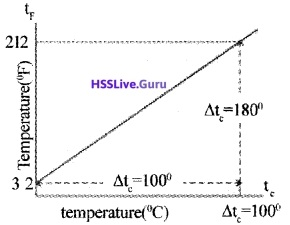If T is temperature in kelvin scale, then
T = tc + 273.15.
A comparison of the three temperature scales is shownIdeal Gas Equation & Absolute Temperature Boyle’s law:
At constant temperature, pressure is inversely proportional to volume.
p α $$\frac{1}{V}$$
PV = constant _______(1)
Charle’s law: At constant pressure, volume is directly proportional to temperature
v α T
$$\frac{V}{T}$$ = constant ______(2)
Combining (1) and (2), we get PV
$$\frac{PV}{T}$$ = constant T
This is called ideal gas law. Generally, the law can be expressed for any quantity of dilute gas asµ is the number of moles in given gas and R is universal gas constant.
The value of R is 8.31 J mol-1 k-1.
Absolute Temperature:
The minimum value of temperature for ideal gas is – 273.15°C (OK). This temperature is called absolute zero. On kelvin scale -273.15°C is taken as zero point.
Note:
The absolute temperature for a gas can be obtained by extrapolating the pressure versus temperature graph as shown below.Thermal Expansion
The change in temperature of a body may change its length, area or volume. The fractional change in dimension [ratio of change in dimension to original dimension] is proportional to change in temperature.

The corresponding proportionally constant is called coefficient of thermal expansion or thermal expansivity. Thermal expansion can be defined as ratio of increase in dimension of body to increase in temperature.

There are different three types of thermal expansion, which are shown in the table given below
Note: (1)
Show that the coefficient of volume expansion for ideal gas is reciprocal of temperature
v = 1/T)
Proof: Ideal Gas Equation isAt constant pressure P∆V = µR∆T ______(2).
Dividing we get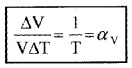Anomalous behavior of water:
Generally volume of liquid increases with temperature .When water is heated, its volume starts to decrease from 0°C and reaches minimum at 4°C. Hence density of water is maximum at 4°C.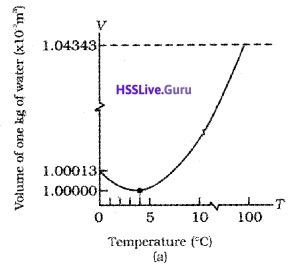Question 1.
Derive the following relations

1. αa = 2αl
2. αv = 3αl

Consider a cube of length ‘l’. Due to the increase in temperature ‘∆T’, length of cube increases by ∆l in all directions.
Coefficient of linear expansion, αl = $$\frac{\Delta \ell}{\ell \Delta \mathrm{T}}$$
1. Increase in area of cube ∆A
= Final area – initial area
= (l + ∆l)2 – l2 = 2 × l × ∆l
[Neglecting ∆l2]
Area expansivityTherefore, αa = 2 . αl.

2. Due to ‘∆T ’ the increase in volume of cube,
∆V = (l + + ∆l)3 – l3
= 3l2∆l2
[Neglecting ∆l2 & ∆l3]Therefore, αv = 3 . αl.Specific Heat Capacity
Heat capacity:
Heat capacity (S) of substance is the quantity of heat required to increase the temperature of whole substance.
If ∆Q is the amount of heat required to increase the temperature by ∆T the heat capacity.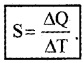Specific heat capacity:
Specific heat capacity of a substance is defined as amount of heat required to increase temperature of unit mass of substance by one unit.
If ∆Q is amount of heat absorbed by substance of mass m and ∆T is change in temperature, then specific heat capacity is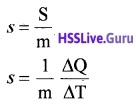The SI unit of specific heat capacity is J Kg-1K-1.
Molar specific heat capacity (C):
Molar specific heat capacity of a substance is the amount of heat required to increase the temperature of 1 mole of substance by one unit.
Its unit is J mol-1 K-1.
If a sample has ‘µ’ moles of substance, then its molar specific heat capacity is given byMolar specific capacity are of two types:

• molar specific capacity at constant volume (CV)
• molar specific heat capacity at constant pressure (CP).Note:

• Water has high specific heat capacity. So it is used as coolant in automobile radiators and as a heater in hot water bags.
• Due to high specific heat capacity of water, land is more warmer than water during daytime.

Calorimetry
Calorimetry means measurement of temperature. Calorimeter is a device used to measure heat. Calorimeter consists of a metallic vessel and a stirrer of same type. The vessel is kept inside a wooden jacket.

The wooden jacket contains insulating mate-rials like glass, wool etc. and hence it prevent heat loss. This jacket has a small opening at top and a thermometer is inserted into this hole.

Change Of State
A transition from one state (solid, liquid or gas) to another state is called change of state. There are four such transitions of state.

 Change of state Name of transition Solid → Liquid Liquid → gas Liquid → solid Solid  → gas (without forming liquid) Melting Vapurization Fusion SublimationDuring change of state, the two different state coexist in thermal equilibrium and temperature remains constant until the completion of change of state.

Melting point:
The temperature at which solid and liquid coexist in thermal equilibrium with each other is called melting point. The melting point decreases with pressure

Boiling point.
The temperature at which liquid and vapour state of substance coexist in thermal equilibrium with each other is called boiling point. The boiling point increases with increase in pressure and it decreases with decrease in pressure.

RegelationTake an ice block. Put a metal wire over the ice block and attach 5 kg. blocks at the two ends of wire as shown. Then we can see that the metal wire passes through the ice block to the other side without splitting it.

Explanation: The melting point of ice just below the wire decreases due to increase in pressure. As ice melts wire passes and refreeze (due to decrease in pressure). This process is called regelation.

Question 2.
Cooking is difficult at high altitude. Why?
At high altitude, pressure is low. Boiling point decreases with decrease in pressure.

Question 3.
For cooking rice pressure cooker is preferred. Why?
In pressure cooker, boiling point of water is increased by increasing pressure. Thus rice can be cooked at high temperature.Question 4.
You might have observed the bubbles of steam coming from bottom of vessel when water is heated. These bubbles disappear as it reaches top of liquid just before boiling and they reach the surface at the time of boiling. Explain the reason?
Just before boiling, the bottom of liquid will be warm and at the top, liquid will be cool. So the bubbles of steam formed at bottom rises to cooler water and condense, hence they disappear. At the time of boiling, temperature of entire mass of water will be 100°C. Now the bubbles reaches top and then escape.

1. Latent Heat:
The amount of heat per unit mass transferred during change of state of substance is called latent heat of substance for the process.
Eg: Latent heat of vaporization (Lv), Latent heat of fusion (Lf).
If ‘m’ is quantity of substance which undergoes change of state and Q is amount of heat required, then latent heat
L = $$\frac{Q}{m}$$
Latent heat is characteristic of substance and it depends on pressure. Its unit is JKg-1.

Question 5.
Draw the temperature versus heat diagram for water. Mark the three phases of water (including its change of state).
Give reasons forthe following

1. The slope of phase line during change of state iszero.
2. The slope of phase line forthe three phases are different.1. During change of state temperature remains constant.
2. Specific heats of different phases are different.Question 6.
Burns from steam are usually more serious than boil-ing water. Why?
Latent heat of vaporization for water is 22.6 × 105J Kg-1 (ie; 22.6 × 105J heat is required to convert 1 kg of water into steam at 100°C). So at 100°C, steam carries 22.6 × 105J. (more heat than water).

Heat Transfer
Heat transfer occurs due to temperature difference. The three modes of heat transfer are

1. conduction
2. convection

1. Conduction:
In conduction, heat transfers between two adjacent parts of a body due to temperature difference. Heat conduction can be considered as time rate of heat flow (heat current). At steady state the time rate of heat flow (H) is proportional to temperature difference ∆T area of cross section (A) and inversely proportional to length of conductor (L).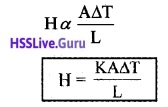K is called thermal conductivity.
Its unit is JS-1m-1K-1 or Wm-1K-1.

Question 7.
Some cooking pots have copper coating on its bottom. Why?
Because of high thermal conductivity of copper, it distributes heat over the bottom of pot very quickly and promotes uniform cooking.

Note: In the house with concrete roof, a layer of insulatiori is made on the ceiling to prevent heat transfer and hence to keep the room cooler.

2. Convection:
In convection, different parts of fluid moves from one point to other. Convection can be natural of forced.
In natural convection when fluid is heated, it expands and becomes less dense. It then rises up and colder part replaces it. This process goes on as a cycle.Question 8.
Explain the reason for sea breeze
During the day, land heats up more quickly than water in lake (due to high specific heat capacity of water). The air on the surface of earth gets heated, expands, becomes less dense and rises up. The colder air (wind) replaces the space created by hot air. It creates a sea breeze. At night the land loses its heat very quickly than water. So water remains more warmer at night.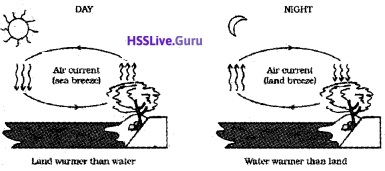Note: In forced convection, material is forced to move by pump or by other physical means. Some examples are cooling system of automobile engines, heart that circulate blood throughout our body.

In radiation, energy is transferred in the form of electromagnetic radiation called heat radiation. Medium is not required for heat transfer. Earth receives energy from sun by means of radiation.

The electromagnetic radiation entitled by a body by virtue of its temperature is called thermal radiation.

Question 9.
The untensils for cooking purpose are blackened at the bottom. Why?
This is to absorb maximum heat from fire and hence to fast up cooking.

Black body:
A black body is one which absorbs radiations of all wavelengths incident on it. When a black body is heated it will emit radiations of all possible wavelengths. The wavelengths emitted by a perfect black body are called black body radiations.
Energy distribution in a black body radiation:Lummer and Pringsheim performed an experiment to study the distribution of energy (among the radiation emitted by a black body) at different temperatures.
Result of experiment:
1. Fora given temperature the energy distribution is not uniform.

2. The energy associated with both longer and shorter wavelength of radiation emitted is small.

3. For each temperature there exists a particular wavelength corresponding to which the energy associated is maximum (λm).

4. This maximum energy carrying wavelength (λm) decreases with an increase in temperature of the black body.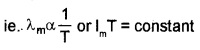5. The area under each curve represents the total energy emitted by the body at a particular temperature.

This area increases with increase of temperature. It is found that area is directly proportional to the fourth power of absolute temperature,
ie. E a T4
Wein’s displacement law:
Wein’s displacement law states that the product of the wavelength corresponding to maximum energy (λm) and the absolute temperature of black body is constant.
ie. λmT = constant
The value of the constant (Wein’s constant) is 2.9 × 10-3mK.
This law explains why the colour of a piece of iron heated in a hot flame first becomes dull red, then reddish yellow and finally white hot.

Wein’s law is useful for estimating the surface temperatures of moon, sun and other stars. If red and blue stars emit radiations of continuous wavelengths, then blue star is hotter than red star.

Stefan’s law states that the total radiant energy emitted persecond from unit area of the surface of a black body is directly proportional to the fourth power of its absolute temperature.
E a T4
E = sT4

Green house effect:
The earth surface is a source of thermal radiation because it absorbs energy received from sun. The wavelength of this radiation lies in the infrared region. But a larger portion of this radiation is absorbed by greenhouse gases, (CO2, CH4, etc).

This heats up the atmosphere. The net result is heating up of earths surface and atmosphere. This is known green house effect.Newtons laws of cooling
According to Newton’s law of cooling the rate of loss of heat is directly proportional to difference of temperature between the body and its surroundings.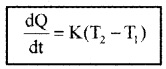T1 is temperature of surrounding medium and T2 is temperature of body. K is constant that depends on nature of surface and area of exposed surface.
Note:

• The law is applicable for small temperature difference.
• For small temperature difference, cooling occurs due to a combination of conduction, convection, and radiation.
• The graph between difference in temperature and time is as shown in figure.